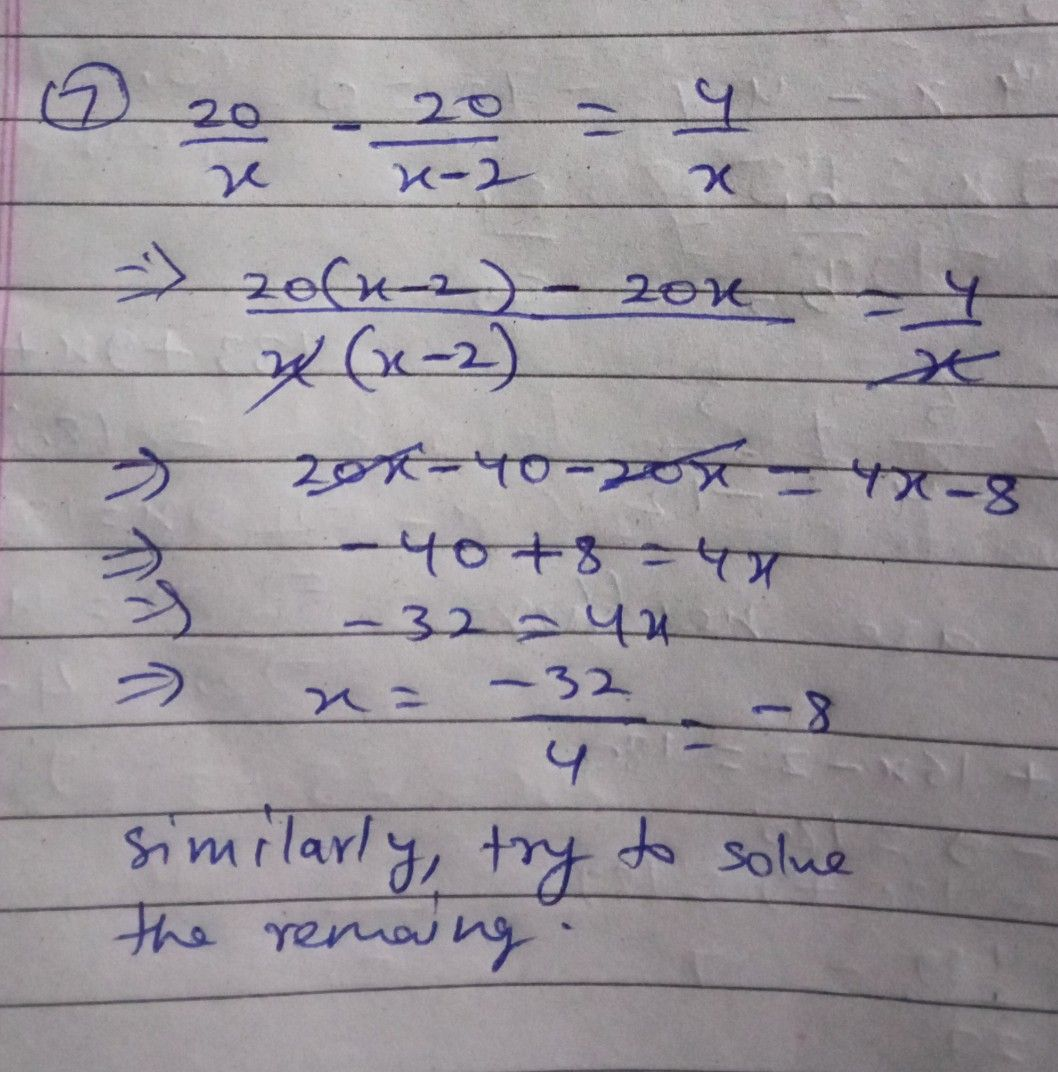Symbol
ProblemLEVEL $2$ $7$ $8$ $\dfrac {20} {x}-\dfrac {20} {x-2}=\dfrac {4} {x}$ $\dfrac {2} {x^{2-x}}=\dfrac {1} {x-1}$ $9$ $10$ $\dfrac {x+32} {x+6}\leq 6$ $\dfrac {x-9} {3x+2}\geq 3$
10th-13th grade
Other
Search count: 135
SolutionQanda teacher - Ankit264